# Schwarzian derivative

(diff) ← Older revision | Latest revision (diff) | Newer revision → (diff)

Schwarz derivative, Schwarzian differential parameter, of an analytic functionof a complex variableThe differential expression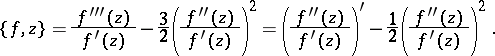It first appeared in studies on conformal mapping of polygons onto the disc, in particular in the studies of H.A. Schwarz .

The most important property of the Schwarzian derivative is its invariance under fractional-linear transformations (Möbius transformations) of the function, i.e. if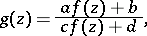then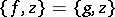. Applications of the Schwarzian derivative are especially connected with problems on univalent analytic functions. For example, ifis a univalent analytic function in the disc, and if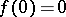,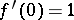, then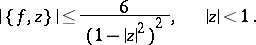Conversely, ifis regular inand if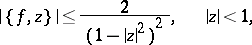thenis a univalent function in, and it is impossible in this case to increase the constant 2.

How to Cite This Entry:
Schwarzian derivative. Encyclopedia of Mathematics. URL: http://encyclopediaofmath.org/index.php?title=Schwarzian_derivative&oldid=14479
This article was adapted from an original article by E.D. Solomentsev (originator), which appeared in Encyclopedia of Mathematics - ISBN 1402006098. See original article